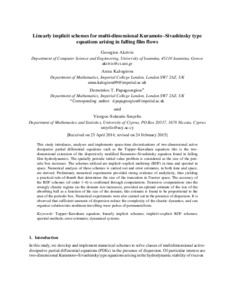# Linearly implicit schemes for multi-dimensional Kuramoto-Sivashinsky type equations arising in falling film flows

Akrivis, Georgios, Kalogirou, Anna, Papageorgiou, Demetrios T. and Smyrlis, Yiorgos-Sokratis (2016) Linearly implicit schemes for multi-dimensional Kuramoto-Sivashinsky type equations arising in falling film flows. IMA Journal of Numerical Analysis, 36 (1). pp. 317-336. ISSN 0272-4979Preview PDF (Accepted manuscript) - Accepted Version Download (1MB) | Preview

## Abstract

This study introduces, analyses and implements space-time discretizations of two-dimensional active dissipative partial differential equations such as the Topper-Kawahara equation; this is the two-dimensional extension of the dispersively modified Kuramoto-Sivashinsky equation found in falling film hydro-dynamics. The spatially periodic initial value problem is considered as the size of the periodic box increases. The schemes utilized are implicit-explicit multistep (BDF) in time and spectral in space. Numerical analysis of these schemes is carried out and error estimates, in both time and space, are derived. Preliminary numerical experiments provided strong evidence of analyticity, thus yielding a practical rule-of-thumb that determines the size of the truncation in Fourier space. The accuracy of the BDF schemes (of order 1-6) is confirmed through computations. Extensive computations into the strongly chaotic regime (as the domain size increases), provided an optimal estimate of the size of the absorbing ball as a function of the size of the domain; this estimate is found to be proportional to the area of the periodic box. Numerical experiments were also carried out in the presence of dispersion. It is observed that sufficient amounts of dispersion reduce the complexity of the chaotic dynamics, and can organize solution into nonlinear travelling wave pulses of permanent form.

Item Type: Article topper-kawahara equation,linearly implicit schemes,implicit-explicit bdf schemes,spectral methods,error estimates,dynamical systems,parabolic equations,analyticity Pure Connector 13 Jan 2017 00:06 22 Oct 2022 02:06 https://ueaeprints.uea.ac.uk/id/eprint/62028 10.1093/imanum/drv011View Item